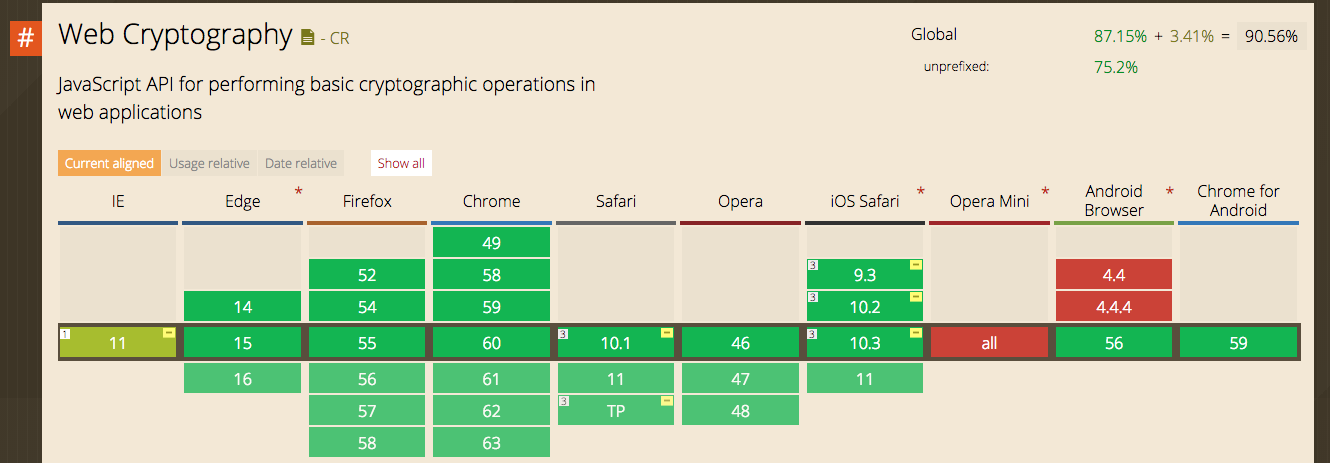# 浏览器环境下 JavaScript 生成随机字符串的科学方法（字母数字）

## window.crypto (Web Cryptography API)

Web Cryptography API 已经是 W3C Recommendation 级别，常见浏览器的近期版本也都已经实现了。使用的时候也可以不用担心兼容性问题。

## crypto.getRandomValues

`crypto.getRandomValues` 是我们用来实现随机字符串的主要方法，这个方法从实现和随机性的角度来说，更加高效、可靠。 10.2.1. The getRandomValues method The getRandomValues method generates cryptographically random values. It must act as follows:

1. If array is not of an integer type (i.e., Int8Array, Uint8Array, Int16Array, Uint16Array, Int32Array, Uint32Array or UInt8ClampedArray), throw a TypeMismatchError and terminate the algorithm.

2. If the byteLength of array is greater than 65536, throw a QuotaExceededError and terminate the algorithm.

3. Overwrite all elements of array with cryptographically random values of the appropriate type.

4. Return array.

Note Do not generate keys using the getRandomValues method. Use the generateKey method instead.

## 科学的随机字符串生成方法

``````let len = 64;
const arr = new Uint8Array(len / 2);
``````

``````window.crypto.getRandomValues(arr);
``````

``````// dec2hex :: Integer -> String
function dec2hex (dec) {
return ('0' + dec.toString(16)).substr(-2);
}

// generateId :: Integer -> String
function generateId (len = 40) {
const arr = new Uint8Array(len / 2);
window.crypto.getRandomValues(arr);
return Array.from(arr, dec2hex).join('');
}
``````